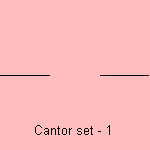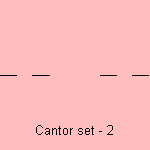Cantor set

fractal

last updated: 2005-01-07

George Cantor (1845-1918) constructed the Cantor set.
The curve is a base motif fractal which uses a line segment as base. The motif is to divide the line segment into three equal parts and replace the middle:The fractal dimension of the Cantor set is equal to log2/log3, what is about 0.6309 1).
The Cantor set can be generalized to a motif where the middle section 1 - 2a is omitted.
Then the fractal dimension is equal to log2/log(1/a).

The Sierpinski curve is a two-dimensional variant on the Cantor set.
The Menger sponge is a three-dimensional variant on the Cantor set.

notes

1) Fractal dimension = log N / log e, where N is the number of line segments and e the magnification.
For the Cantor set: N=2, e=3.